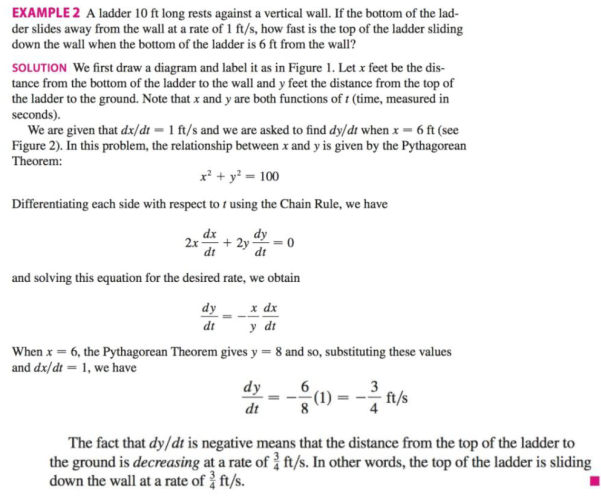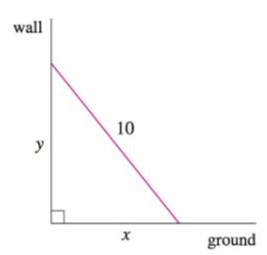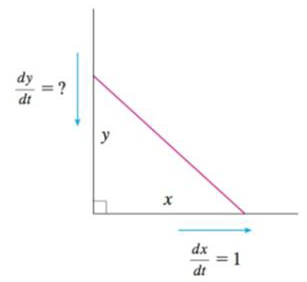Chapter 3.9, Problem 34E

Chapter
Section
Textbook Problem

According to the model we used to solve Example 2, what happens as the top of the ladder approaches the ground? Is the model appropriate for small values o f y?EXAMPLE 2FIGURE 1FIGURE 2To determine

To find: Find the validity of the model when top of the ladder approaches to the ground.

Explanation

Given:

A ladder which is rests against a vertical wall and the on the horizontal ground.

Suddenly the bottom of the ladder slides away from the ground at a rate of 1 ft/s.

Formula used:

(1) Chain rule: dydx=dydududx

(2) Pythagorean Theorem.

Calculation:

Let us assume that L be the length of the ladder and x  be the horizontal distance from the wall to the lower end of the ladder, and y be the vertical distance from the ground to the top end of the ladder as shown in the Figure 1 given below.

Since the ladder slides away from the wall so the distance x become increases and y decreases.

Therefore x and y are function of the time t

Now from the Pythagorean Theorem.

x2+y2=L2

Differentiate with respect to the time t .

ddt[x2+y2]=ddt[L2]2xdxdt+2ydydt=0[</

Still sussing out bartleby?

Check out a sample textbook solution.

See a sample solution

The Solution to Your Study Problems

Bartleby provides explanations to thousands of textbook problems written by our experts, many with advanced degrees!

Get Started

Find more solutions based on key concepts